# How to☝️ Make a Mortgage Calculator in Google Sheets

Whenever people purchase a home, many may need to take out a mortgage loan. If you find yourself considering a mortgage on a new home, you may find questions like these filling your mind: What will be the equated monthly installment (EMI) for the mortgage loan I am taking? What would be the ideal tenure for me to take this loan? What will be the EMI based on varying interest rates?

If you do find yourself wondering about such things, then this article may be just the thing to help!

In this article, we will see how to create your very own mortgage calculator so you can figure out the answers to all questions raised above. Let’s dive in!

## How to Make a Mortgage Calculator (Step by Step)

Step 1: Start by adding some of the essential information about your mortgage to your sheet, such as the Principal Mortgage Amount, Annualized Interest Rate, and Tenure in Years.

Suppose you want to take out a loan of \$600,000 at 7% interest with a tenure of 20 years. Fill in these details under Google Sheets across cells B2:C4 as shown below: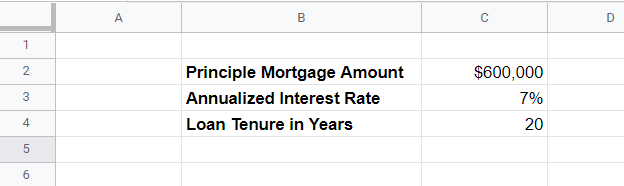You will need these details in order to create your own mortgage calculator.

Step 2: Across cells B8:F8, type the following descriptions related to each attribute you will have in your mortgage calculator: Months, Monthly EMI, Monthly Principal Amount, Monthly Interest, and Outstanding Principal.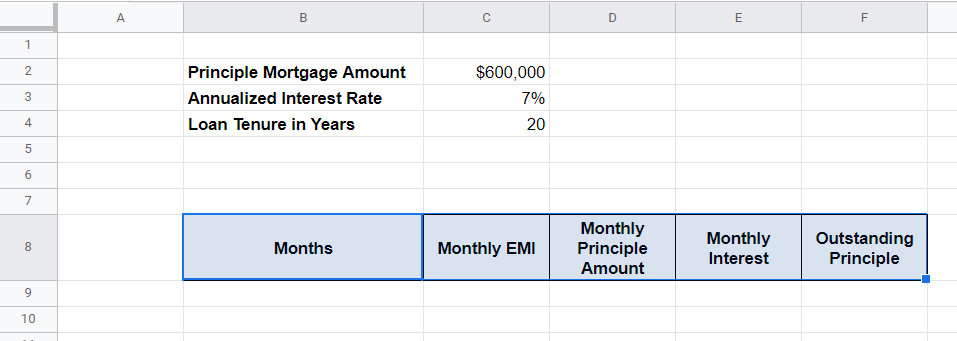These attributes are pretty straightforward:

• Months – Represents the number of months in your loan.
• Monthly EMI – Represents the amount you pay every month.
• Monthly Principal Amount – Represents the contribution toward the principal amount in your monthly EMI.
• Monthly Interest – Represents the interest amount you pay every month.
• Outstanding Principle – Represents the outstanding principal amount you have after each payment.

Now let’s take a look at building the Google Sheets mortgage calculator formula step by step.

Step 3: For the Months column, type the first month’s value as 1 in cell B9, then use the formula B9+1 in cell B10 to return the following month’s value. Drag this formula downward across the rows until you have labeled 240 months (20 years * 12 months per year).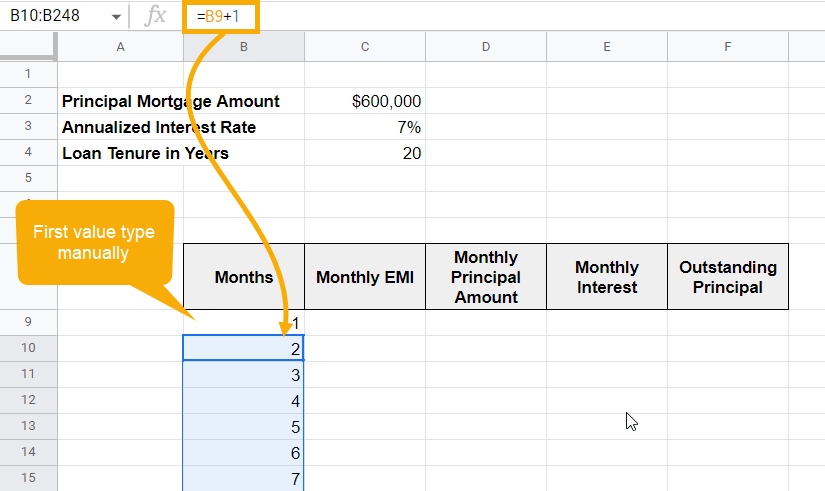Before filling in the Monthly EMI column, we will first capture the Monthly Principal Amount and Monthly Interest. Then we can use them both to calculate the Monthly EMI.

Step 4: In cell D9, copy and paste the following formula to calculate the Monthly Principal Amount:

=PPMT(\$C\$3/12,B9,\$C\$4*12,-\$C\$2)

Drag the formula across the rows to capture the Monthly Principal Amount in the entire column D for 240 months. It should look like the example shown below: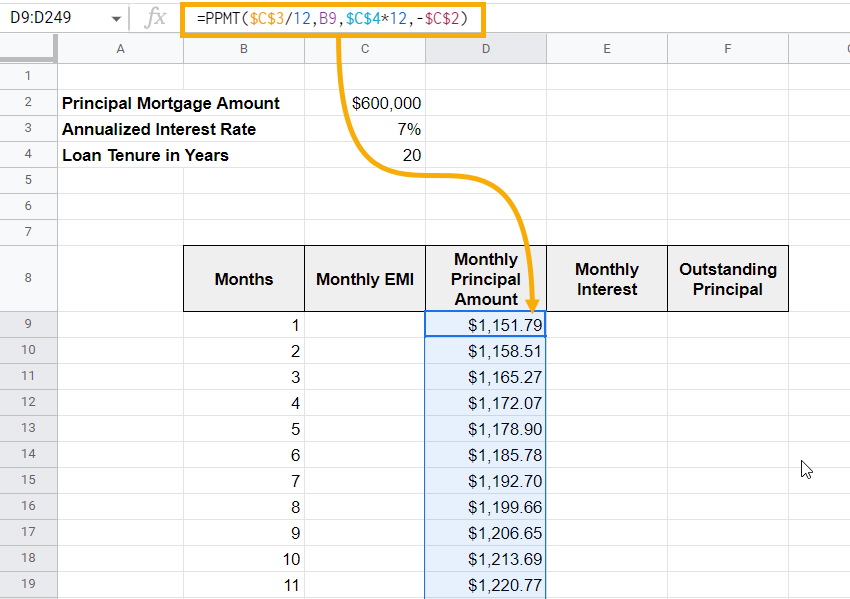### Formula Explanation

The first argument for the PPMT formula asks for the rate of interest. In this case, the value comes from cell C3 (Annualized Interest Rate). To convert it to a monthly interest rate, we divided it by 12. Also, it is mandatory to fix the reference for this cell (using dollar signs) to keep it from changing when you drag the formula down.

The second argument for the formula is the time period—what month it is in the life of the loan—which is in cell B9.

Next, specify the number of periods for the entire loan. Since you are holding the loan for 20 years, use the absolute reference of cell C4. However, you do need to convert it from years to months, so multiply it by 12. Again, make sure you fix the reference for the cell.

Finally, mention the present value, which is your Principal Mortgage Amount from cell C2. Since you have borrowed that amount, it should be mentioned as a negative value. Moreover, it will not change across the calculation, so you should apply an absolute reference to it.

Step 5: Now, to calculate the Monthly Interest, use the formula shown below and paste it into cell E9.

=IPMT(\$C\$3/12,B9,\$C\$4*12,-\$C\$2)

Note that it is the same formula, just with IMPT instead of PPMT. Drag this formula down, and you will see the Monthly Interest in column E.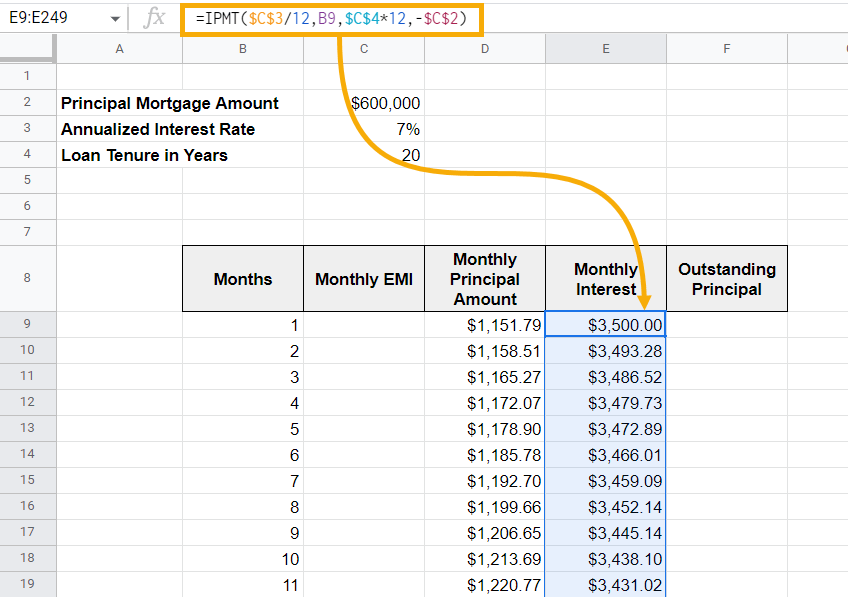The IPMT function works similarly to PPMT. The only difference is that it captures the interest amount whereas PPMT captures the Principal amount from the total EMI paid.

Pro Tip: “P” in PPMT stands for Principal Amount, and “I” in IPMT stands for Interest Amount. This is also one of the ways to remember these two formulas.

Since we now know the Monthly Principal Amount and Monthly Interest Amount, it is easy to calculate the Monthly EMI. You just need to sum these two components to get the Monthly EMI.

Step 6: In cell C9, use the formula =D9+E9 to get the Monthly EMI value. Drag it down to get the EMI amount for each month. Generally, this component of a mortgage calculator is the same for all the months. You can cross-check it from the screenshot below as well.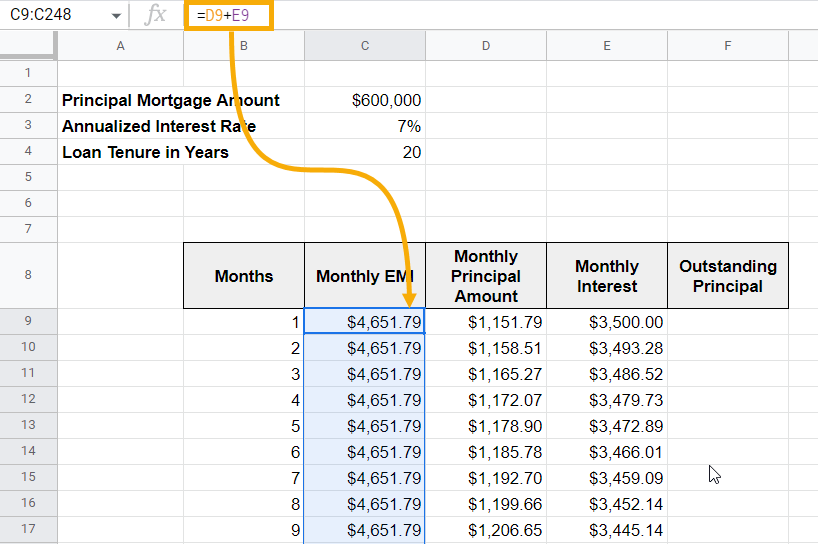Note: Typically, when you first take out a loan, the Interest Rate contributes more to the monthly EMI, and the Principal Amount contributes less. Gradually, this changes. As your loan progresses, the Principal Amount makes up more of the Monthly EMI.

Finally, in column F, the Outstanding Principal Amount can be captured.

Step 7: In cell F9, type the formula =C2D9 to calculate the Outstanding Principal Amount after the first month.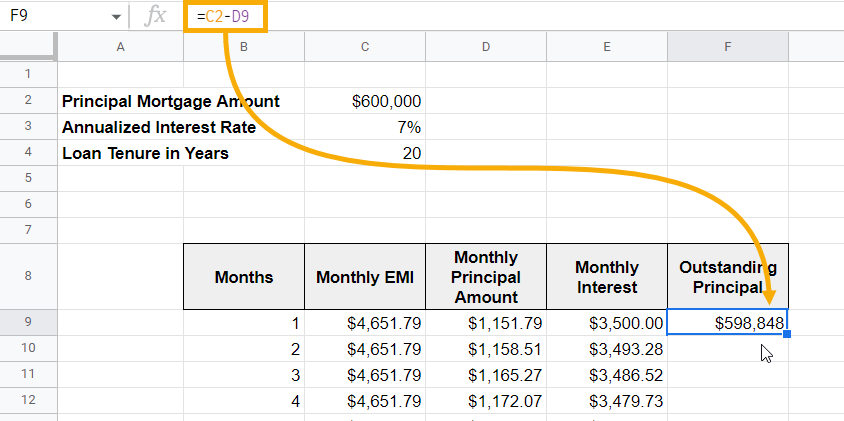This formula subtracts the first month’s Principal Amount from the Principal Mortgage Amount of \$600,000.

The second month’s Outstanding Principal will be the first month’s Outstanding Principal value subtracted by the second month’s Principal Amount.

Step 8: In cell F10, use the formula =F9D10 to calculate the following Outstanding Principal Amount. Drag this formula downward through the rest of the list to calculate the Outstanding Principal Amount for the remaining months.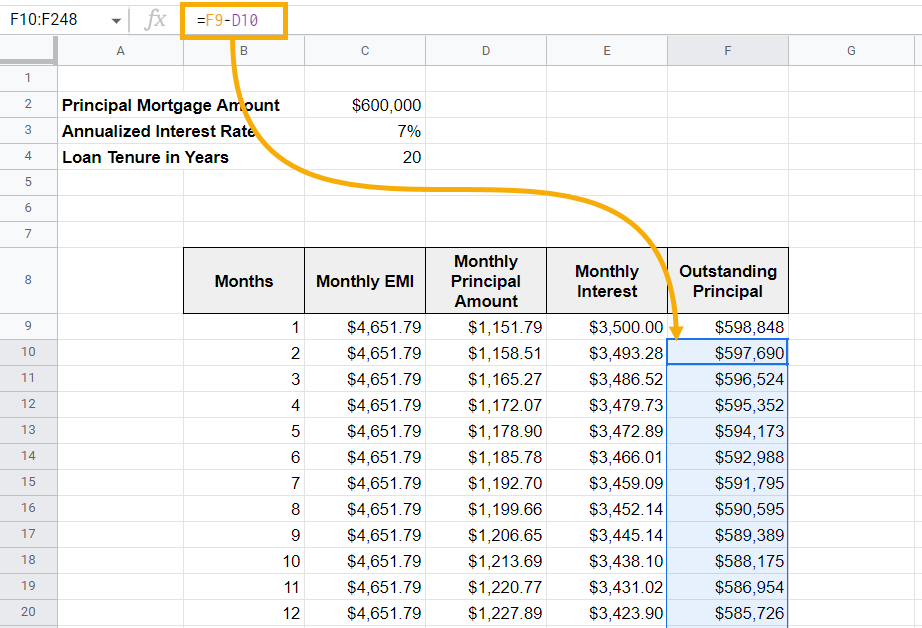## FAQs

### How do I calculate mortgage payments in Google Sheets?

If you follow our step-by-step guide, you can calculate your mortgage payments pretty quickly in Google Sheets. The calculator is free to use, and you can make a copy of it for yourself and use it to calculate different amounts, interest rates, and periods (loan tenure) as well.

### Does Google Sheets have a mortgage calculator?

So far, Google Sheets doesn’t have a readily available mortgage calculator. However, you can use the one we have created or check out many of the other free-to-use mortgage calculators created by other Google Sheets users.